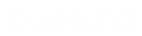Feedback

• Contents

# Dialer agent statistics

These statistics summarize the activity of all Dialer agents.

Abandon Rate (by calls)

The percentage of total Dialer calls handled by all agents which were classified as abandons.

Abandon Rate (by contacts)

The percentage of total Dialer calls handled by all agents which were contacts and were classified as abandons.

Abandon Rate (by detections)

The percentage of Dialer calls handled by all agents which reached a live party and were classified as abandons.

Average Break Time

The average amount of time all agents have spent on break.

Average Dialer Talk Time

The average amount of time all agents have spent on each Dialer call.

Average Idle Time

The average amount of time all agents have spent idle.

Average Non-Dialer Talk Time

The average amount of time all agents have spent on each non-Dialer call.

Average Talk Time

The average amount of time all agents have spent on each call.

Contact Rate

The percentage of total Dialer calls handled by all agents which were classified as contacts.

Contacts Per Hour

The number of Dialer calls resulting in a contact which all agents have handled per hour.

Dialer Calls

The number of Dialer calls all agents have completed.

Idle Periods

The number of times all agents have been idle.

Logged In Time

How long the agents have been logged into the campaign.

Non-Dialer Calls

The number of non-Dialer calls all agents have completed.

Percent Break Time

The percentage of all agents's time which has been spent on break.

Percent Dialer Talk Time

The percentage of all agents's time which has been spent on Dialer calls.

Percent Idle Time

The percentage of all agents's time which has been spent idle.

Percent non-Dialer Talk Time

The percentage of all agents's time which has been spent on non-Dialer calls.

Stage

The stage the agents are currently in.

Station

The station the agents are logged into.

Status

The agents's current status.

Successes Per Hour

The number of successful Dialer calls all agents handled per hour.

Successes Rate (by calls)

The percentage of total Dialer calls handled by all agents which were classified as successes.

Successes Rate (by contacts)

The percentage of Dialer calls handled by all agents which were contacts and were classified as successes.

Time in Stage

How long the agents have been in the current stage.

Time in Status

How long the agents have been in the current status.

Total Abandons

The number of calls all agents have completed that were classified as abandons.

Total Break Time

The total amount of time all agents have spent on break.

Total Breaks

The number of times all agents have been on break.

Total Contacts

The number of calls all agents have completed that were classified as contacts.

Total Detections

The number of calls all agents have completed that were detected as a live speaker.

Total Dialer Talk Time

The total amount of time all agents have spent on connected Dialer calls.

Total Emails

The total number of emails sent.

Total Idle Time

The total amount of time all agents have spent idle.

Total Non-Dialer Talk Time

The total amount of time all agents have spent on connected non-Dialer calls.

Total SMS Messages

The total number of SMS messages sent.

Total Successes

The number of calls all agents have completed that were classified as successes.

Total Talk Time

The total amount of time all agents have spent on Dialer and non-Dialer calls.